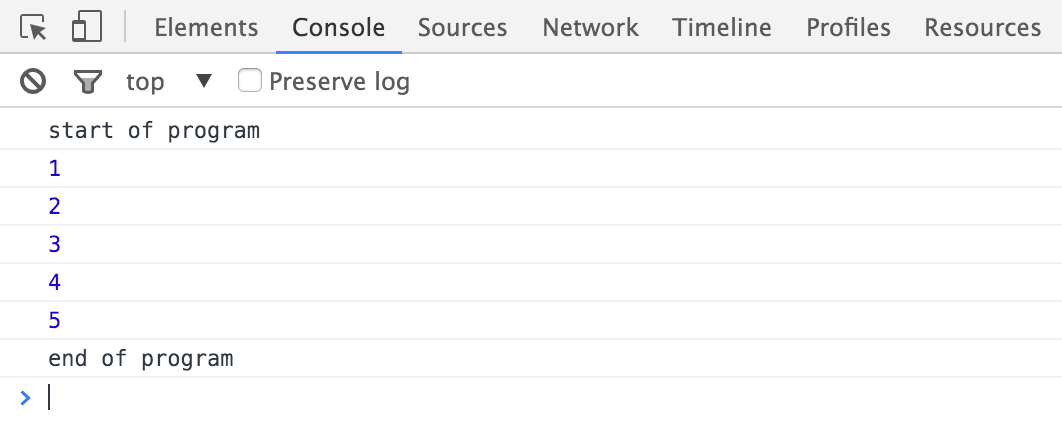Last updated on 2/11/20

## Repeat statements

In this chapter, we'll look at how to execute code on a repeating basis!

### Introduction

If you wanted to write code that displayed numbers between 1 and 5, you could do it with what you've already learned:

``````console.log(1);
console.log(2);
console.log(3);
console.log(4);
console.log(5);``````

This is pretty tiresome though and would be much more complex for lists of numbers between 1 and 1000, for example. How can you accomplish the same thing more simply?

JavaScript lets you write code inside a loop that executes repeatedly until it's told to stop. Each time the code runs, it's called an iteration.

### "While" loops

A  `while` loop lets you repeat code while a certain condition is true.  Here's a sample program written with a `while` loop.

``````console.log("start of program");
var number = 1;
while (number <= 5) {
console.log(number);
number++;
}
console.log("end of program");``````In your `chapter-4`  folder, add the above file contents to test it out.

You'll use the following syntax to write a  `while` loop.

``````while (condition) {
// code to run while the condition is true
}``````

Before each loop iteration, the condition in parentheses is evaluated to determine whether it's true or not. The code associated with a loop is called its body!

• If the condition's true, the code in the  `while`  loop's body runs. Afterward, the condition is re-evaluated to see if it's still true or not. The cycle continues!

• If the condition is false, the code in the loop stops running or doesn't run.

### "For" loops

You'll often need to write loops with conditions that are based on what happens in the loop body, like in our example. JavaScript offers another loop type to account for this: the `for` loop.

#### How it works

Here's the proper  `for`  loop syntax:

``````for (initialization; condition; final-expression) {
// code to run when condition is true
}``````

This is a little more complicated than  `while` loop syntax:

• Initialization only happens once, when the code first kicks off.

• The condition is evaluated once before the loop runs each time. If it's true, the code runs. If not, the code doesn't run.

• The final expression is evaluated after  the loop runs each time. It's often used to update a counter variable, as we saw in the `while` loop example.

The variable used during initialization, condition, and the final expression is often called a counter.

``````for (var counter = 1; counter <= 5; counter++) {
console.log(counter);
}``````

### Common errors

#### Infinite loops

The main risk with `while` loops is a producing an infinite loop, meaning the condition is always true, and the code runs forever. This will crash your program! For example, let's say you forget a code line that increments the `number` variable. If you try to open the page in Chrome, the browser will freak out, and you'll strain your system.

``````var number = 1;
while (number <= 5) {
console.log(number);
// The number variable is always 1, so the loop will run each time...forever.
}``````

#### Manipulating a "for" loop counter

Imagine that you accidentally modify the loop counter in the loop body.

``````for (var counter = 1; counter <= 5; counter++) {
console.log(counter);
counter++; // the counter variable changes within the loop body
}``````

Each time the loop runs, the counter variable is incremented twice: once in the body and once in the expression after the loop runs. When you're using a `for` loop, you'll almost always want to omit anything to do with the counter from the body of your loop. Just leave it in that first line!

### Which loop should I use?

`For` loops are great because they include the notion of counting by default, which avoids the problem of infinite loops. However, it means you have to know how many times you want the loop to run as soon as you write your code. For situations where you don't already know how many times the code should run, `while` loops make sense. As for a good `while` loop example, let's imagine a user will enter letters over and over until they enter X:

``````var letter = "";
while (letter !== "X") {
letter = prompt("Type any letter or X to exit:");
console.log(letter);
}``````

You can't know how many times it'll take for the user to enter X, so  `while`  is generally good for loops that depend on user interaction. Ultimately, choosing which loop to use depends on context. All loops can be written with  `while`  , but if you know in advance how many times you want the loop to run,  `for`  is the best choice.